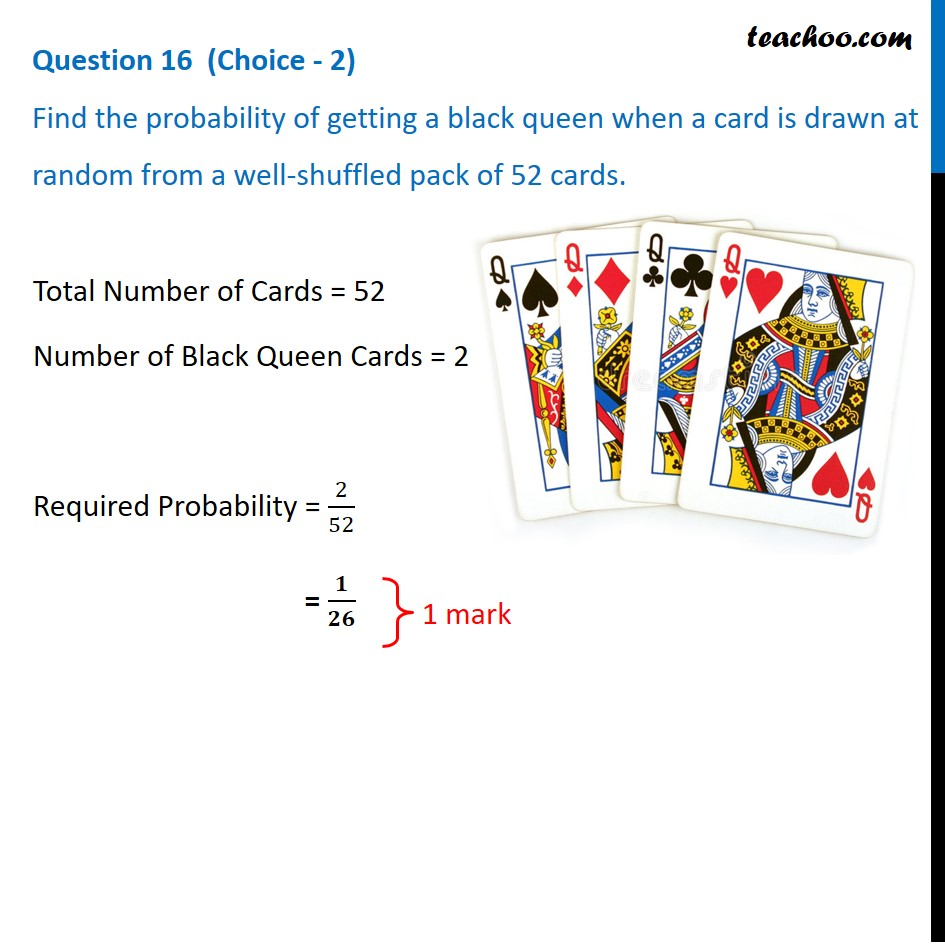## Find the probability of getting a black queen when a card is drawn at random from a well-shuffled pack of 52 cards.1. Class 10
2. Solutions of Sample Papers for Class 10 Boards
3. CBSE Class 10 Sample Paper for 2021 Boards - Maths Standard

Transcript

Question 16 (Choice - 2) Find the probability of getting a black queen when a card is drawn at random from a well-shuffled pack of 52 cards. Total Number of Cards = 52 Number of Black Queen Cards = 2 Required Probability = 2/52 = 𝟏/𝟐𝟔

CBSE Class 10 Sample Paper for 2021 Boards - Maths Standard

Class 10
Solutions of Sample Papers for Class 10 Boards